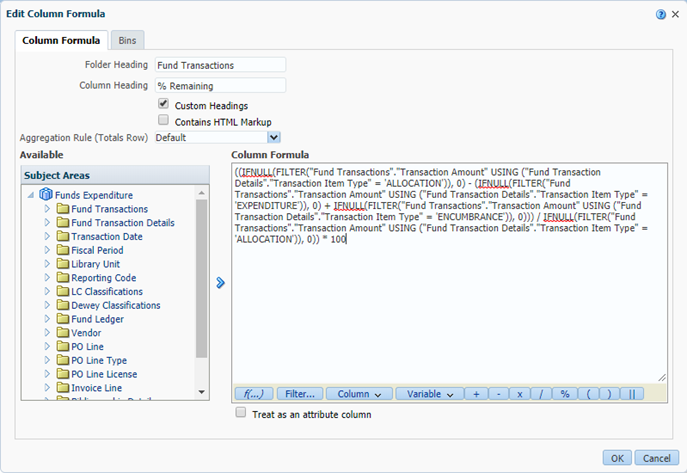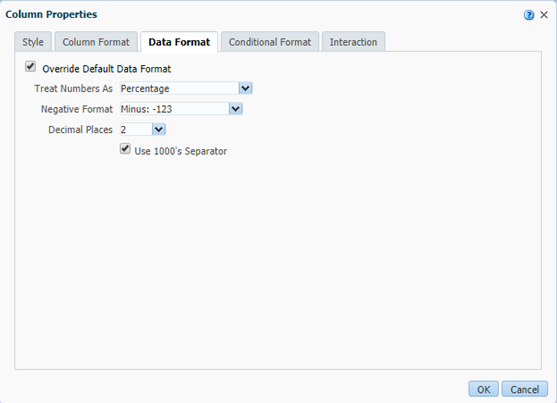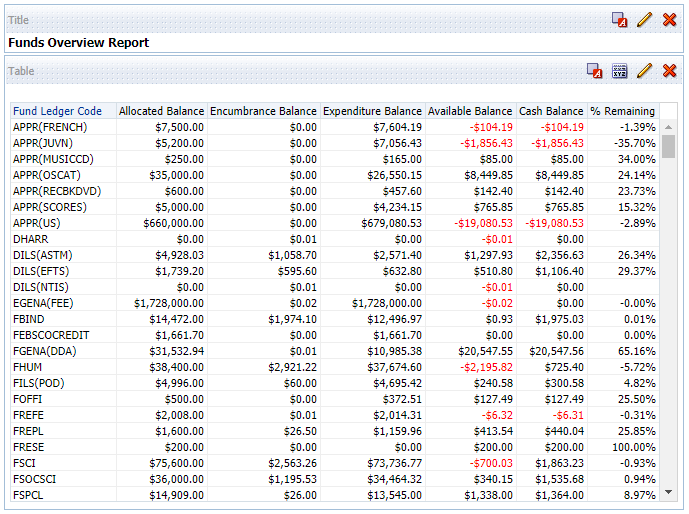• # How to calculate in Analytics the % remaining of a fund considering both expenditures and encumbrances

Created By: Mike Rogers
Created on: 4/15/2019

It is possible to calculate the percentage (%) remaining of a fund considering both expenditures and encumbrances.  The formula should be this:

((IFNULL(FILTER("Fund Transactions"."Transaction Amount" USING ("Fund Transaction Details"."Transaction Item Type" = 'ALLOCATION')), 0) - (IFNULL(FILTER("Fund Transactions"."Transaction Amount" USING ("Fund Transaction Details"."Transaction Item Type" = 'EXPENDITURE')), 0) + IFNULL(FILTER("Fund Transactions"."Transaction Amount" USING ("Fund Transaction Details"."Transaction Item Type" = 'ENCUMBRANCE')), 0))) / IFNULL(FILTER("Fund Transactions"."Transaction Amount" USING ("Fund Transaction Details"."Transaction Item Type" = 'ALLOCATION')), 0)) * 100

You should be able to copy & paste the above formula as-is into the Edit Formula field, then click OK:Note also that you should change the Data Format to treat Numbers as percentages for this column.  That can be found under “Column Properties” for that column, then the Data Format tab:I usually take the Decimal Places out to two but you can use whatever you want here.

Using the formula will give output like this (the % Remaining column is using the above formula):